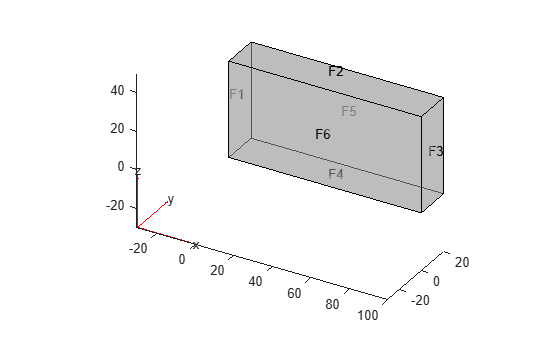Main Content

## View, Edit, and Delete Boundary Conditions

### View Boundary Conditions

A PDE model stores boundary conditions in its `BoundaryConditions` property. To obtain the boundary conditions stored in the PDE model called `model`, use this syntax:

`BCs = model.BoundaryConditions;`

To see the active boundary condition assignment for a region, call the `findBoundaryConditions` function.

For example, create a model and view the geometry.

```model = createpde(3); importGeometry(model,'Block.stl'); pdegplot(model,'FaceLabels','on','FaceAlpha',0.5)```Set zero Dirichlet conditions for all equations and all regions in the model.

`applyBoundaryCondition(model,'dirichlet','Face',1:6,'u',[0,0,0]);`

On face 3, set the Neumann boundary condition for equation 1 and Dirichlet boundary condition for equations 2 and 3.

```h = [0 0 0;0 1 0;0 0 1]; r = [0;3;3]; q = [1 0 0;0 0 0;0 0 0]; g = [10;0;0]; applyBoundaryCondition(model,'mixed','Face',3,'h',h,'r',r,'g',g,'q',q);```

View the boundary condition assignment for face 3. The result shows that the active boundary condition is the last assignment.

```BCs = model.BoundaryConditions; findBoundaryConditions(BCs,'Face',3)```
```ans = BoundaryCondition with properties: BCType: 'mixed' RegionType: 'Face' RegionID: 3 r: [3x1 double] h: [3x3 double] g: [3x1 double] q: [3x3 double] u: [] EquationIndex: [] Vectorized: 'off' ```

View the boundary conditions assignment for face 1.

`findBoundaryConditions(BCs,'Face',1)`
```ans = BoundaryCondition with properties: BCType: 'dirichlet' RegionType: 'Face' RegionID: [1 2 3 4 5 6] r: [] h: [] g: [] q: [] u: [0 0 0] EquationIndex: [] Vectorized: 'off' ```

The active boundary conditions assignment for face 1 includes all six faces, though this assignment is no longer active for face 3.

### Delete Existing Boundary Conditions

To remove all the boundary conditions in the PDE model called `pdem`, use `delete`.

`delete(pdem.BoundaryConditions)`

To remove specific boundary conditions assignments from `pdem`, delete them from the `pdem.BoundaryConditions.BoundaryConditionAssignments` vector. For example,

```BCv = pdem.BoundaryConditions.BoundaryConditionAssignments; delete(BCv(2))```

Tip

You do not need to delete boundary conditions; you can override them by calling `applyBoundaryCondition` again. However, removing unused assignments can make your model more concise.

### Change a Boundary Conditions Assignment

To change a boundary conditions assignment, you need the boundary condition’s handle. To get the boundary condition’s handle:

• Retain the handle when using `applyBoundaryCondition`. For example,

```bc1 = applyBoundaryCondition(model,'dirichlet', ... 'Face',1:6, ... 'u',[0 0 0]);```
• Obtain the handle using `findBoundaryConditions`. For example,

```BCs = model.BoundaryConditions; bc1 = findBoundaryConditions(BCs,'Face',2)```
```bc1 = BoundaryCondition with properties: BCType: 'dirichlet' RegionType: 'Face' RegionID: [1 2 3 4 5 6] r: [] h: [] g: [] q: [] u: [0 0 0] EquationIndex: [] Vectorized: 'off'```

You can change any property of the boundary conditions handle. For example,

```bc1.BCType = 'neumann'; bc1.u = []; bc1.g = [0 0 0]; bc1.q = [0 0 0]; bc1```
```bc1 = BoundaryCondition with properties: BCType: 'neumann' RegionType: 'Face' RegionID: [1 2 3 4 5 6] r: [] h: [] g: [0 0 0] q: [0 0 0] u: [] EquationIndex: [] Vectorized: 'off'```

Note

Editing an existing assignment in this way does not change its priority. For example, if the active boundary condition was assigned after `bc1`, then editing `bc1` does not make `bc1` the active boundary condition.

## SupportGet trial now